Refer to our Texas Go Math Grade 1 Answer Key Pdf to score good marks in the exams. Test yourself by practicing the problems from Texas Go Math Grade 1 Lesson 11.4 Answer Key Add or Subtract.

Unlock the Problem

Nicole sees 16 turtles on the beach. Some turtles swim away. There are 9 turtles still on the beach. How many turtles swim away?What information am I given?
_________ turtles
_________ swim away
__________ turtles still on the beach

Plan

What is my plan or strategy?
I can _____________

Solve

Show how you solve the problem.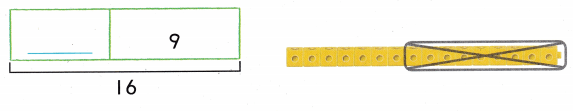16 turtles ______ swim away
9 turtles still on the beach
Seven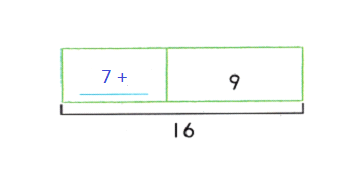Given,
There are sixteen turtles on the beach, some of them swim away
Now there are nine turtles still on the beach
By subtracting nine from sixteen we get seven,
So, seven number of turtles swim away.

HOME CONNECTION • Your child made o model to visualize the problem. The model helps your child see what part of the problem to find.

Model and Draw

Make a model to solve. Use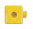to help you.Question 1.
There are 4 rabbits in the field. Some more rabbits come. Now there are 12 rabbits. How many rabbits come to the field?4 rabbits __________ rabbits come
12 rabbits in the field
Given,
There are 4 rabbits in the field, Some rabbits come to join the field
Now there are total of 12 rabbits in the field
We know that,
By subtracting four from twelve we get eight,
So eight number of rabbits come to join the field.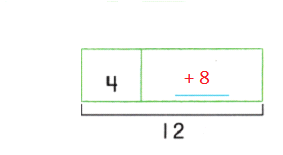Question 2.
There are 14 birds in a tree. Some birds fly away. There are q birds still in the tree. How many birds fly away?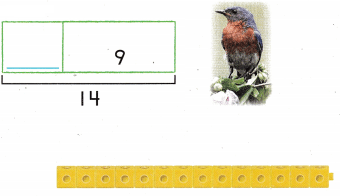14 birds __________ birds fly away
9 birds still in the tree
Given,
There are fourteen birds in a tree and some birds fly away.
Now there are nine birds still in the tree.
We know that,
By subtracting nine with fourteen we get five,
So five number of birds fly away from the tree.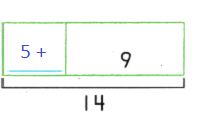Math Talk
Mathematical Processes

Explain how to find the unknown number.

Share and Show

Make a model to solve. Use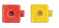to help you.

Question 3.
There are 20 ducks in the pond. Then 10 ducks swim away. A How many ducks are still in the pond?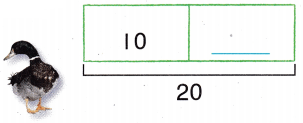20 ducks
10 swim away
_________ ducks still in the pond
Given,
There are twenty ducks in the pond,
Ten ducks in the pond swim away,
By subtracting ten from twenty then we get ten,
So there are ten ducks still in the pond.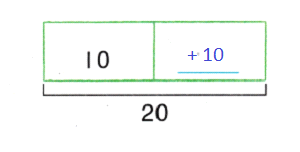Problem Solving

Question 4.
H.O.T. 3 eagles land in the trees. Now 12 eagles are in the trees. How many eagles were in the trees to start?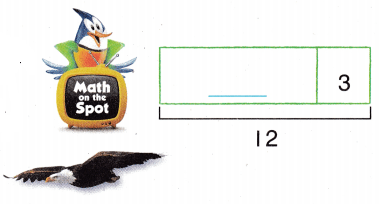________ eagles
3 eagles land
12 eagles in the trees
Given,
Three eagles landed on the tree,
Now there are twelve eagles on the tree,
By subtracting three from twelve we get nine,
So there are nine eagles to start to fly.Question 5.
H.O.T. Multi-Step 8 squirrels are in the park. The same number of squirrels join them. How many squirrels are ¡n the park now?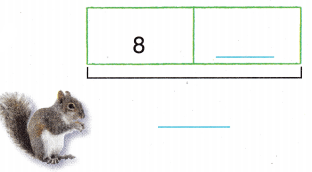8 squirrels __________ squirrels join them ___________ squirrels in the park
Given,
There are eight squirrels in the park,
Same number of squirrels join the park,
By adding eight with eight we get sixteen
That means, there are eighteen squirrels in the park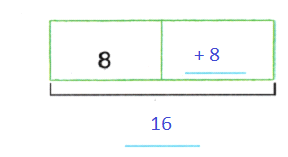Use objects to solve the problem. Choose the correct answer.

Question 6.
There are 6 children at the big tree. More children come to the tree. Now there are 14 children. How many children come to the tree?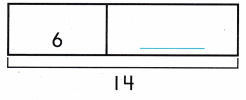(A) 9
(B) 6
(C) 8
Given,
There are six children at the big tree, more children came to the tree
Now there are fourteen children at the big tree
By subtracting six from fourteen we get eight
So eight children came to the tree to joinQuestion 7.
Use Diagrams There are 15 birds in the tree. 7 birds are blue. The other birds are brown. How many birds are brown?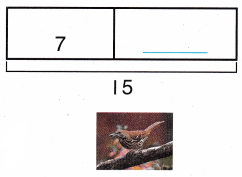(A) 8
(B) 6
(C) 7
Given,
There are fifteen birds in the tree,
Seven of them are blue andd the remaining birds are brown,
By subtracting seven from fifteen we get eight,
So there are eight number of brown birds in the treeQuestion 8.
There are 4 more blue birds than red birds. There are 12 blue birds. How many red birds are there?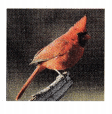(A) 9
(B) 16
(C) 8
Given,
There are four more blue birds than red birds
There are total of twelve blue birds
By subtracting four from twelve we get eight
So there are eight red birds

Question 9.
Texas Test Prep There are 13 fish in the tank. Some fish swim behind the rock. There are 8 fish in front of the rock. How many fish are behind the rock?
(A) 4
(B) 6
(C) 5
Given,
There are thirteen fishes in the tank, some fishes are behind the rock
There are eight fishes in front of the rock,
By subtracting, eight from thirteen we get five,
So there are five fishes behind the rock.

### Texas Go Math Grade 1 Lesson 11.4 Homework and Practice Answer Key

Make a model to solve.

Question 1.
There are 13 frogs by the pond. 6 frogs hop away. How many frogs are still by the pond?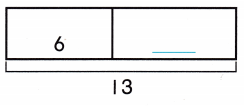13 frogs
6 hop away
__________ frogs still by the pond
Given,
There are thirteen frogs by the pond,
Six frogs hop away,
By subtracting six from thirteen we get seven,
So there are seven frogs still by the pond.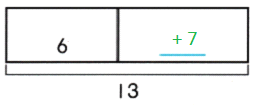Question 2.
Ginny blows up 9 balloons. Now there are 18 balloons. How many balloons were there before?___________ balloons before
9 balloons
18 balloons now
Given,
Ginny blows up nine ballons
Now there are eighteen ballons,
By subtracting nine from eighteen we get nine,
There were nine ballons before.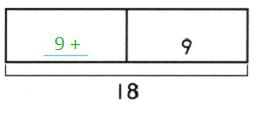Problem Solving

Question 3.
Multi-Step 6 friends play ball in the park. The same number of friends join them. How many friends are in the park now?6 friends
friends join them
________ friends now
Given,
There are six friends playing ball in the park,
The same number of friends joined them
By adding six with six we get twelve,
So there are total of twelve friends in the park.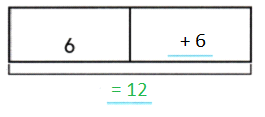Lesson Check

Question 4.
There are 9 desks in the room. The teacher brings in more desks. Now there are 12 desks. How many desks did the teacher bring in?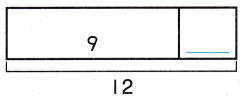(A) 4
(B) 6
(C) 3
Given,
There are 9 desks in the room,
Teacher brings in more desks,
Now there are twelve desks in the rooms,
So by subtracting three from twelve we get three,
So it means, three more desks were brought by the teacher.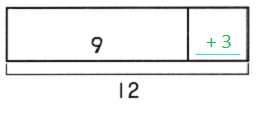Question 5.
A house has 9 more small windows than big windows. The house has 13 windows. How many big windows does it have?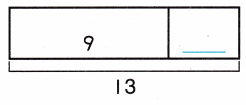(A) 4
(B) 5
(C) 6
Given,
There are thirteen windows, nine of them are small windows
By subtracting nine from thirteen we get four,
So there are four big windows.Question 6.
7 worms are in the garden. 6 more worms crawl over. How many worms are in the garden now?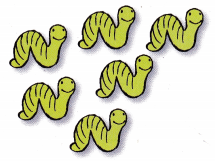(A) 14
(B) 12
(C) 13
Given,
There are seven worms in the garden,
Six more worms crawl over in the garden,
By adding seven with six we get thirteen,
So there are total of thirteen worms in the garden.

Question 7.
There are 16 birds in a tree. Some birds fly away. There are 9 birds left in the tree. How many birds flew away?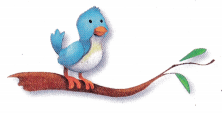(A) 9
(B) 7
(C) 8